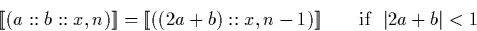Next: Division Up: Normalisation' Previous: Signed binary (mantissa, exponent)

### Dyadic (mantissa, exponent) normalisation'

Dyadic (mantissa, exponent) normalisation' is more complex than the signed binary case. This is because the dyadic digits at the start of the stream can become very small indeed without ever being exactly zero. There are two obvious approaches:

The simplest method is to just remove dyadic zero digits from the head of the mantissa. It is possible, however, that a mantissa representing a very small value which could be normalised' is ignored because one of the early digits are small but non-zero.

The second approach is to add the first digit of the mantissa stream with half the second. If the absolute value of this sum is less than or equal to a half, the first two digits of the mantissa may be replaced by the digit representing twice this sum and the exponent decremented.Martin Escardo
5/11/2000